# mtext Function in R (5 Examples)

In this article you’ll learn how to write text to the margins of a graphic using the mtext function in R programming.

The content of the page looks as follows:

Let’s start right away:

## Creation of Example Data

The data below is used as basement for this R tutorial:

```data <- data.frame(x = 1:7,        # Create example data frame
y = 7:1)
data                               # Print example data frame```As you can see based on Table 1, our example data is a data frame having seven rows and two integer columns.

As next step, we can plot our data:

`plot(data)                         # Draw plot without additional text`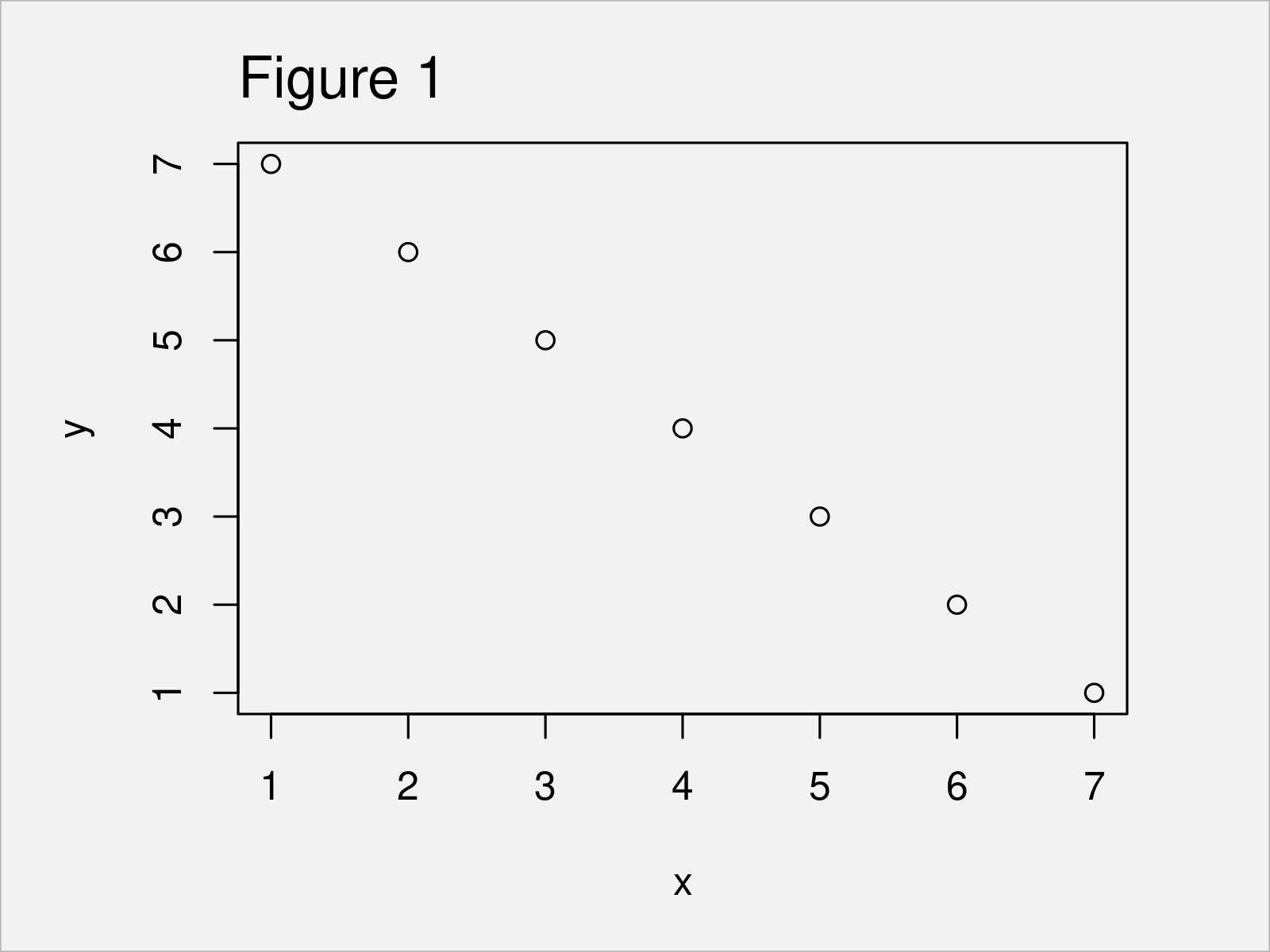As shown in Figure 1, the previously shown code has created a Base R scatterplot without any additional text elements.

## Example 1: Basic Application of mtext() Function

Example 1 shows how to use the mtext function to one of the four margins of the current figure region.

More precisely, we’ll add the text element “This is my text!” to the top (i.e. side = 3) of our plot:

```plot(data)                         # Draw plot
mtext("This is my text!",          # Add text to margins of plot
side = 3)```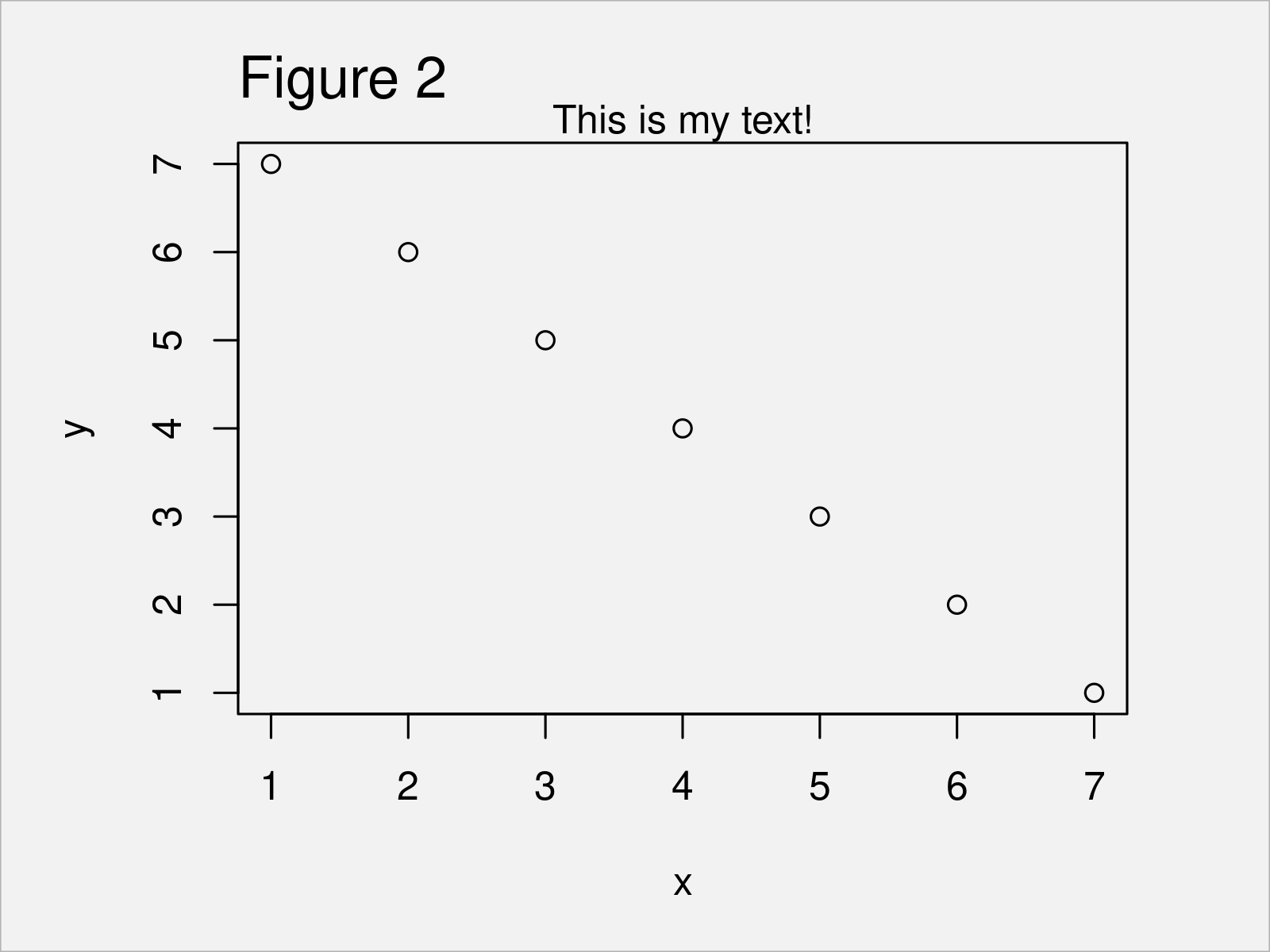In Figure 2 it is shown that we have drawn a text element at the top of our scatterplot by executing the previous code.

## Example 2: Change Side of Plot where Text Should be Added

This example shows how to annotate a text element on the right side of a graph.

For this, we have to set the side argument to be equal to 4:

```plot(data)                         # Draw plot
mtext("This is my text!",          # Add text to margins of plot
side = 4)```In Figure 3 you can see that we have created a new plot with text element on the right side using the previous R programming code.

If we would like to move the text element to the bottom of the graphic, we would have to specify side = 1; and if we would like to switch to the left side, we would have to specify side = 2.

## Example 3: Move Text Inside or Outside of Plot

This example demonstrates how to adjust the vertical positioning of a text element.

For this, we can use the line argument as shown below:

```plot(data)                         # Draw plot
mtext("This is my text!",          # Add text to margins of plot
side = 3,
line = - 2)```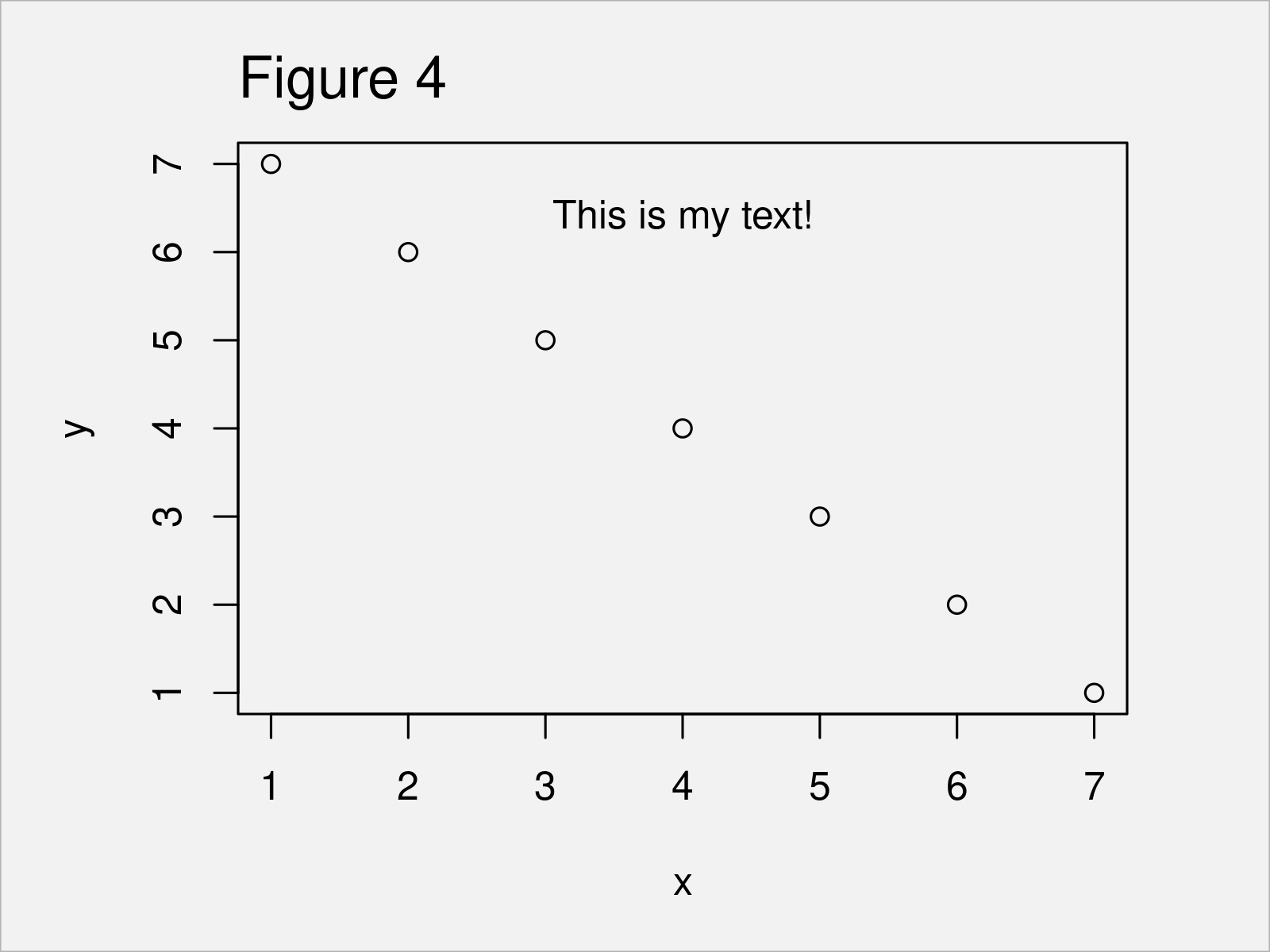## Example 4: Change Size of Text

In Example 4, I’ll demonstrate how to increase the size of the font using the cex argument:

```plot(data)                         # Draw plot
mtext("This is my text!",          # Add text to margins of plot
side = 3,
cex = 2)```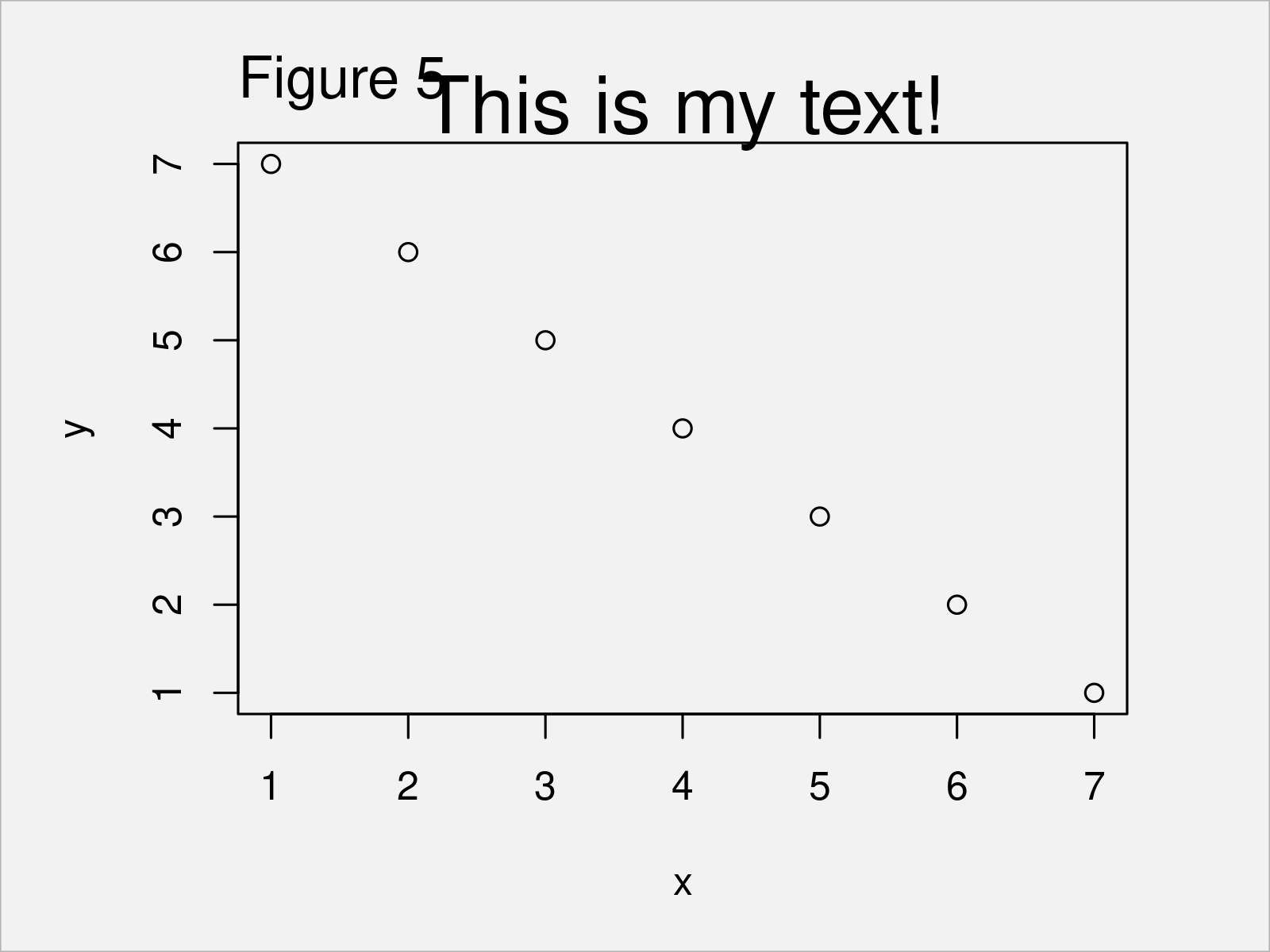The larger the value specified to the cex argument, the larger is the text.

## Example 5: Change Color of Text

In this example, I’ll explain how to modify the color of the text.

For this, we can use the col argument as shown below:

```plot(data)                         # Draw plot
mtext("This is my text!",          # Add text to margins of plot
side = 3,
col = "red")```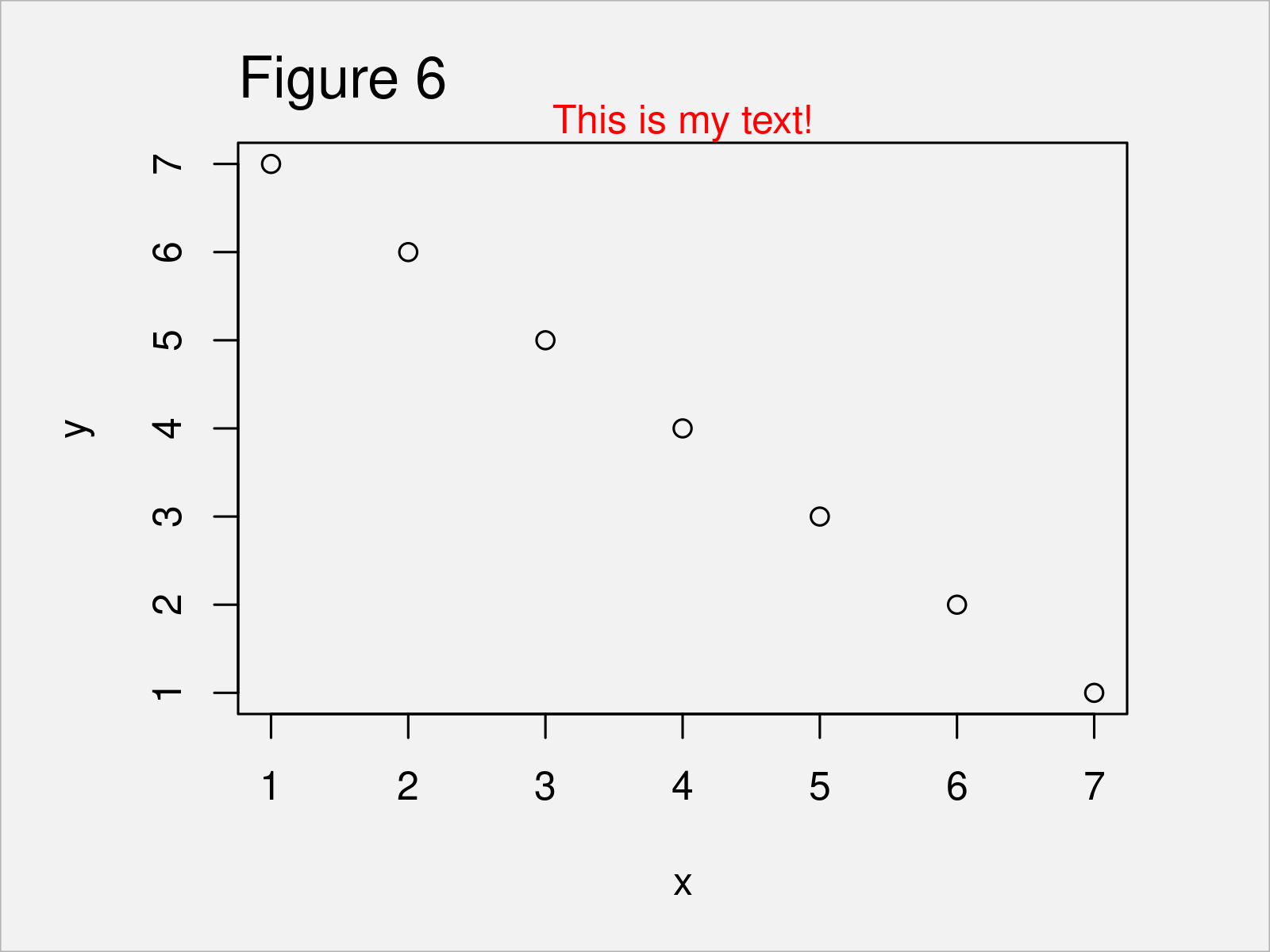## Video & Further Resources

If you need more explanations on the contents of this tutorial, you may want to have a look at the following video on my YouTube channel. I’m explaining the R programming codes of this tutorial in the video:

Please accept YouTube cookies to play this video. By accepting you will be accessing content from YouTube, a service provided by an external third party.If you accept this notice, your choice will be saved and the page will refresh.

Furthermore, you may want to read the other articles on my homepage. Some interesting articles are listed below.

To summarize: This article has illustrated how to add text elements to the margins of a graphic using the mtext function in R programming.

In the present tutorial, we have applied the mtext command to write text into the margins of a Base R scatterplot. However, please note that we could also use this function to annotate text to other types of graphs such as barplots, histograms, line charts, and boxplots.